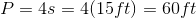# ISEE Upper Level Math : How to find the perimeter of a square

## Example Questions

### Example Question #1 : Squares

Sideshown below in squareis equal to 17.5 inches. What is the perimeter of?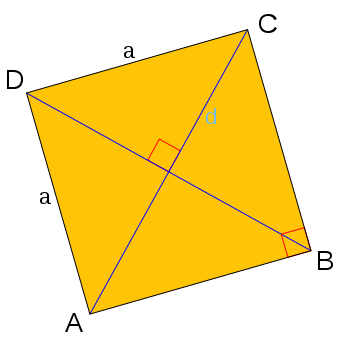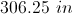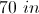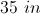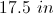Cannot be determinedExplanation:

The perimeter of a quadrilateral is the sum of the length of all four sides. In a square, each side is of equal length. Thus, the perimeter is the length of a side (given) times 4.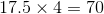### Example Question #2 : Squares

If the area of a square is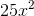, what is the perimeter?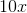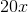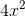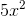Explanation:

If the area of a square is, then the length of one side will be equal to the square root of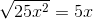The perimeter is equal to 4 times the length of one side.

This gives us: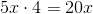### Example Question #3 : Squares

If one of the edges has a length of 6 inches, what is the perimeter of one side of the box?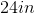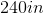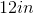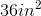Explanation:

If one of the edges has a length of 6 inches, what is the perimeter of one side of the box?

To find perimeter of a square, simply multiply the side length by 4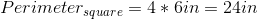### Example Question #4 : Squares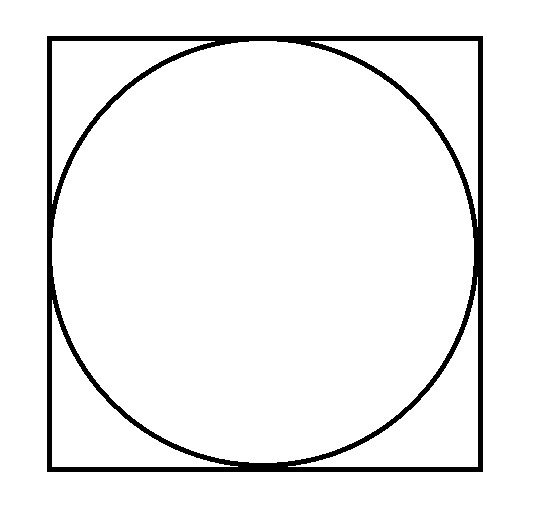In the above diagram, the circle is inscribed inside the square. The circle has circumference 30. What is the perimeter of the square?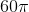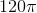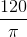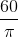Explanation:

Call the diameter of the circle. The length of each side of the square also is equal to this.

The diameter of the circle is equal to its circumference divided by, so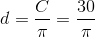.

The perimeter of the square is four times this sidelength, so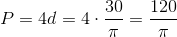.

### Example Question #5 : Squares

Find the perimeter of a square with a width of 4cm.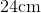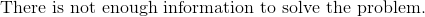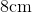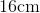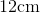Explanation:

To find the perimeter of a square, we will use the following formula: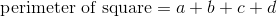where a, b, c, and d are the lengths of the sides of the square.

Now, we know the width of the square has a length of 4cm.  Because it is a square, all sides are equal.  Therefore, all sides are 4cm.

Knowing this, we can substitute into the formula.  We get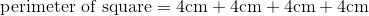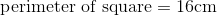### Example Question #6 : Squares

While out walking, you find a strange, square-shaped piece of metal. If the side length of the piece is 26 inches, what is the perimeter of the square?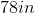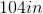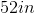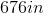Explanation:

While out walking, you find a strange, square-shaped piece of metal. If the side length of the piece is 26 inches, what is the perimeter of the square?

To find the perimeter of a square, simply multiply the side length by 4. We can do this because perimeter is the distance around the outside of a shape, and squares have 4 equal sides.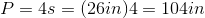### Example Question #7 : Squares

Find the perimeter of a square with a width of 18in.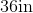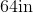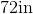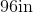Explanation:

To find the perimeter of a square, we will use the following formula: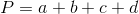where a, b, c, and d are the lengths of the sides of the square.

Now, we know the width of the square is 18in. Because it is a square, all sides are equal. Therefore, all sides are 18in.

So, we get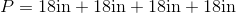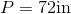### Example Question #8 : Squares

Find the perimeter of a square with a side length of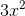.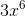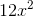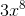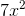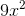Explanation:

A square has 4 equal sides.

Write the formula for the perimeter of a square.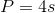Substitute the side length.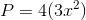Evaluate the terms on the right.

The answer is:### Example Question #9 : Squares

Your new friend has a very small, square-shaped dorm room. She tells you that it is only 225 square feet. Assuming this is true, what is the perimeter of her room?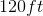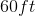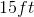Not enough information to solve the problemExplanation:

Your new friend has a very small, square-shaped dorm room. She tells you that it is only 225 square feet. Assuming this is true, what is the perimeter of her room?

So, we need to find the perimeter of a square. First, we need to find the side length.

Let's begin with our formula for the area of a square: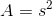where s is our side length and A is our area.

With this formula, we can solve for our side length by plugging in our area and square rooting both sides.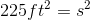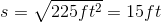Now, we are close but not quite done. We need to multiply our side length by 4, because a square always has 4 equal sides.